# Measures of central tendency: how to use them to give clarity to your data?

If you are constantly working with data, you need to know what measures of central tendency are. They will become your best allies when it comes to making that final report of metrics or project status that you need so much to know how the objectives were met.

We know that there are times when we must deal with a large amount of information, we may have a large number of data, numbers and variables and we do not always know the best way to summarize them. this is where measures of central tendency come into play!

According to Deloitte, “the best data strategies are generally tailored to the needs of the organization”, and that’s why before you jump into calculating, the first thing to keep in mind is your objectives and what information you are looking for in the data analysis.

In this note we will see all about measures of central tendency, what they are, how they are measured and what we can use them for, plus some examples so that the next time you feel overwhelmed by many numbers and data, you will know perfectly how to analyze them.

pick your data tables and let’s get started!

## what are measures of central tendency?

Measures of central tendency are statistical parameters that report the center of distribution of the sample or statistical population. in other words, it is a number that is located towards the center of the distribution of the values of a series of observations or measurements, in which the data set is located.Source: Unsplash

## what are measures of central tendency for?

Measures of central tendency aim to define where the typical average element or number is located in the group or series of data. for example, if I want to know what is the average number of cart abandonment in my e-commerce in a month, I can define it using the mode, which means the most frequent selection.

In the same way, measures of central tendency serve us to compare data and interpret the results obtained from a series of data. thus, what we can determine is the difference between one value and another value. For example, the number of times the cart was left in a given month with respect to the other.

On the other hand, measures of central tendency and averages help us to discard figures outside the range, i.e., anomalies, and thus, work with a series of clean and orderly information. In this case, we could determine whether this or that strategy helped us to reduce abandoned carts.Source: Pexels

## what are the measures of central tendency?

In this section we will see what are the main measures of central tendency, and how to calculate and interpret them correctly so that the decision making in your administrative or marketing strategies are 100% informed .

According to Economipedia, there are three main types of measures of central tendency:

mean
median
mode

### 1. Mean

The mean is the most commonly used measure of central tendency.It is the average value of a set of numerical data. It is calculated by adding the total of the set of values divided by the total number of values, i.e., the sample size. It is expressed in the following mathematical form: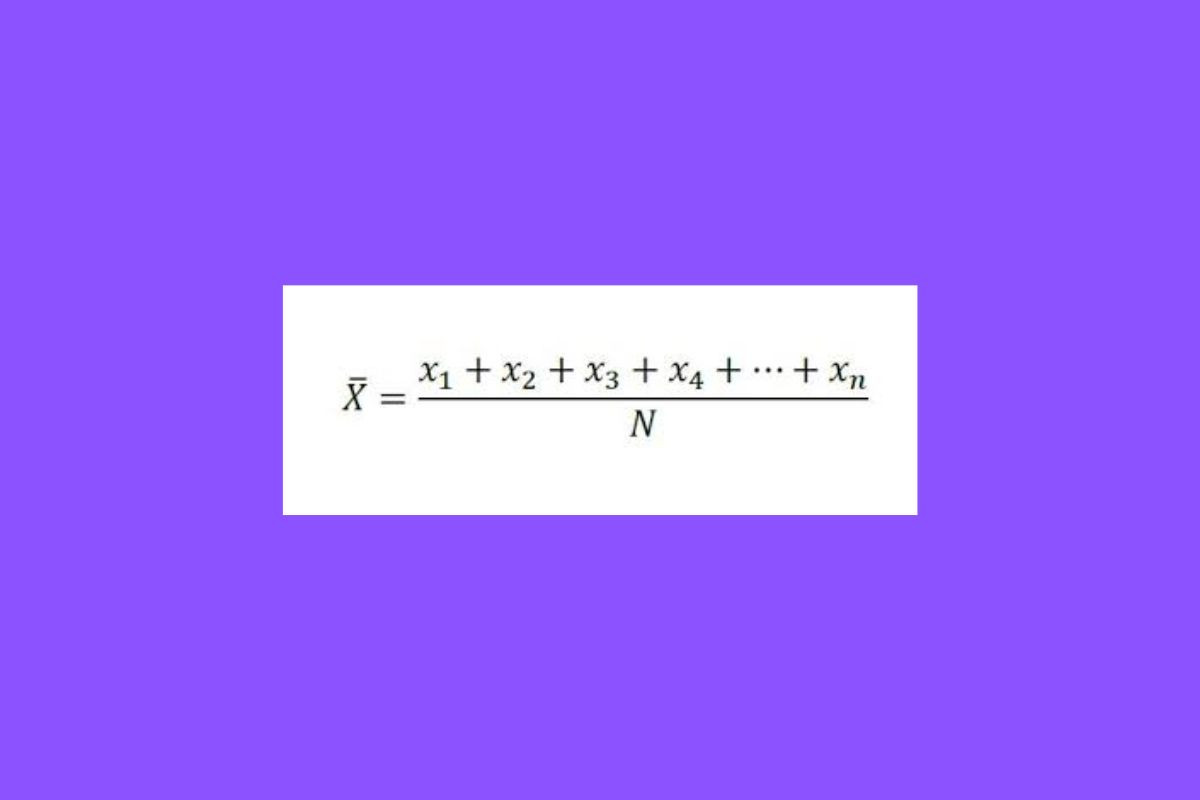need a little more guidance in understanding this measure of central tendency? where x is the value of observation or number i; N the total number of observations or sample size.

how do you calculate the mean?

Suppose you want to determine the average of the number of daily sales in 8 days, and the data in your excel table are: 7,9,10,8,8,6,8,9,7

The average is calculated as follows:

Sample sum = 7+9+10+8+8+6+8+8+9+7=64

Sample size (N) = 8

Mean = 64/8 = 8

As a result, we can say that your business has an average of 8 sales per day in 8 days.

### 2. Median

The next measure of central tendency is the median. by median we mean the statistic that exactly halves the same number of values on one side and on the other.

To calculate the median, what must be done is to “halve” the sample. To do this, it is necessary to group the data from smallest value to largest value, leaving the same number of values on one side as on the other. It is different from the mean as it gives us the average position of the data set.

The mathematical formula for this measure of central tendency is as follows: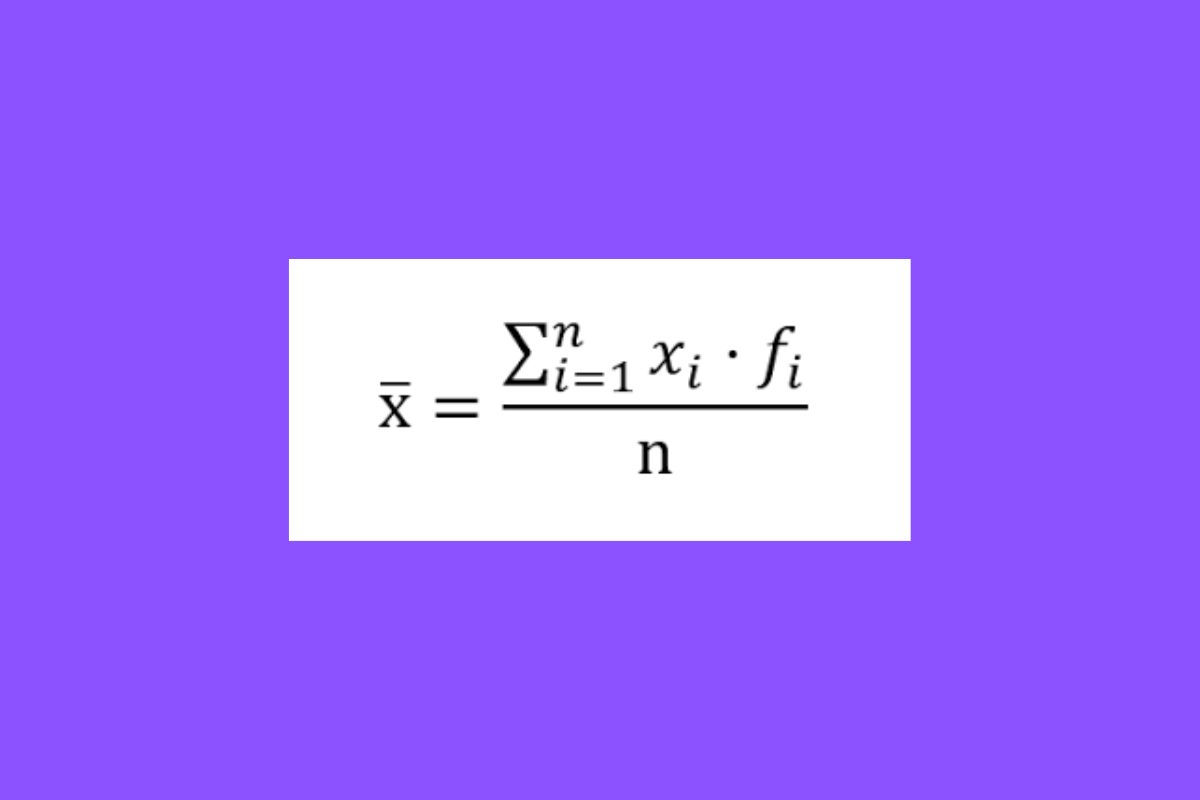how is the median calculated?

Suppose there are two distinct groups of customers you want to target with your next strategy, but for that you want to know what the median sales point is between the two groups, regardless of their distinction:

The first group consists of 8 customers responsible for the sales we saw above: 7,9,10,8,8,6,8,9,7
The second group consists of 11 customers and their sales are very similar to those of the first group but there are 3 more students: 7,9,10,8,6,8,9,9,7,6,10,9

What is important here is that the first group has an even number of customers and the second has an odd number.

Therefore, when the number of observations is even, as it is in the first group, the median is calculated as follows:

The first thing to do is to order the data from smallest to largest: 7,9,10,8,6,8,8,9,7 → 6,7,7,7,8,8,8,9,9,9,10
Then eliminate the values from both sides in the direction of the center: 6,7,7,7,8,8,8,9,9,10

In this case, the midpoint is both values of 8 so the median is 8.

When the number of observations is odd, the same procedure is repeated. In the case of the second group, the median is:

7,9,10,8,6,8,9,7,6,10,9 → 6,6,7,7,8,8,9,9,910,10

6,6,7,7,8,8,9,9,9,10,10

The result is the central value. In the second group with 11 customers, the average or median is 8.

As you can imagine, this is just an example of central tendency measure and surely in your case you have a pile of data to compare, so it will be convenient that you learn how to use the median function in Excel, thanks to this information processor the calculations will be even simpler.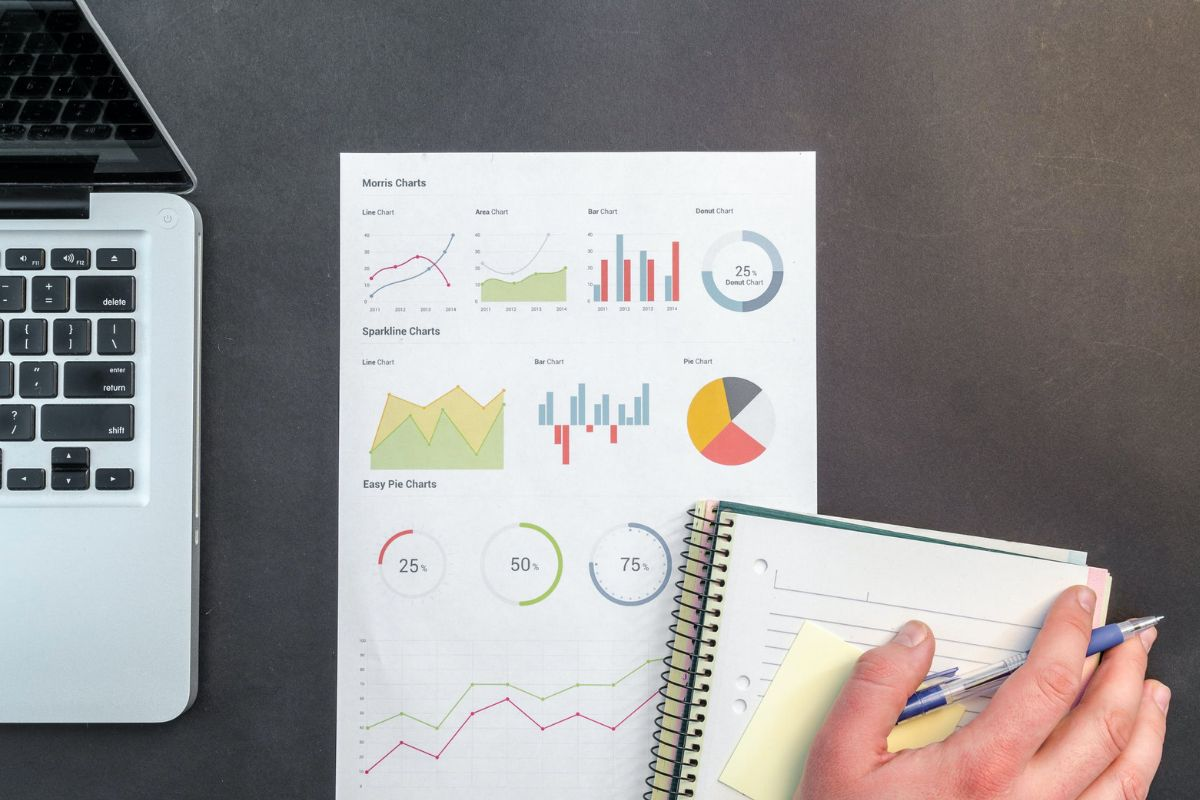Source: Pexels

### 3. Mode

Finally, we come to the mode. This measure of central tendency is simply the most repeated value in the statistical sample or population. Although there is a mathematical formula, the easiest thing to do is to determine which value is the most repeated.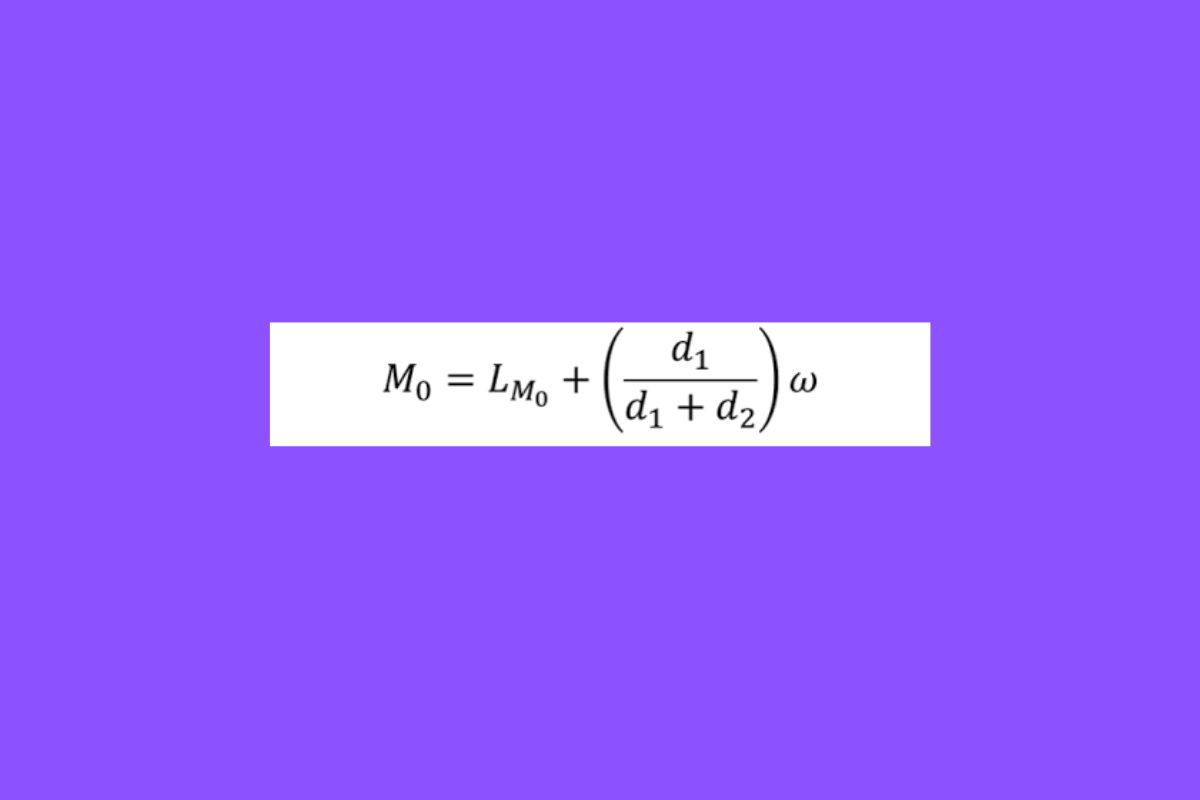Imagine that you are about to launch your own textile line and you need to know the average measurements of the target audience you are aiming at, for that you do a survey and it turns out that the heights of a group of 8 people are:

1.75m, 1.78m, 1.64m, 1.82m, 1.75m, 1.68m, 1.75m, 1.88m

In this case, the mode is 1.75m because it is repeated three times, the largest number in the group.

Unlikethe mean and median, the mode can also be calculated for non-numerical observations. For example, the same group was asked what their favorite food is and they answer the following:

Pizza, tacos, hamburgers, pasta, pizza, tacos, pizza, pizza, sushi

In this case, the trend is pizza.

### Range

Finally, there is the range. although this is not a measure of central tendency, it is useful to see the difference between the smallest and the largest value. It is calculated by simply obtaining the difference between the largest and smallest value.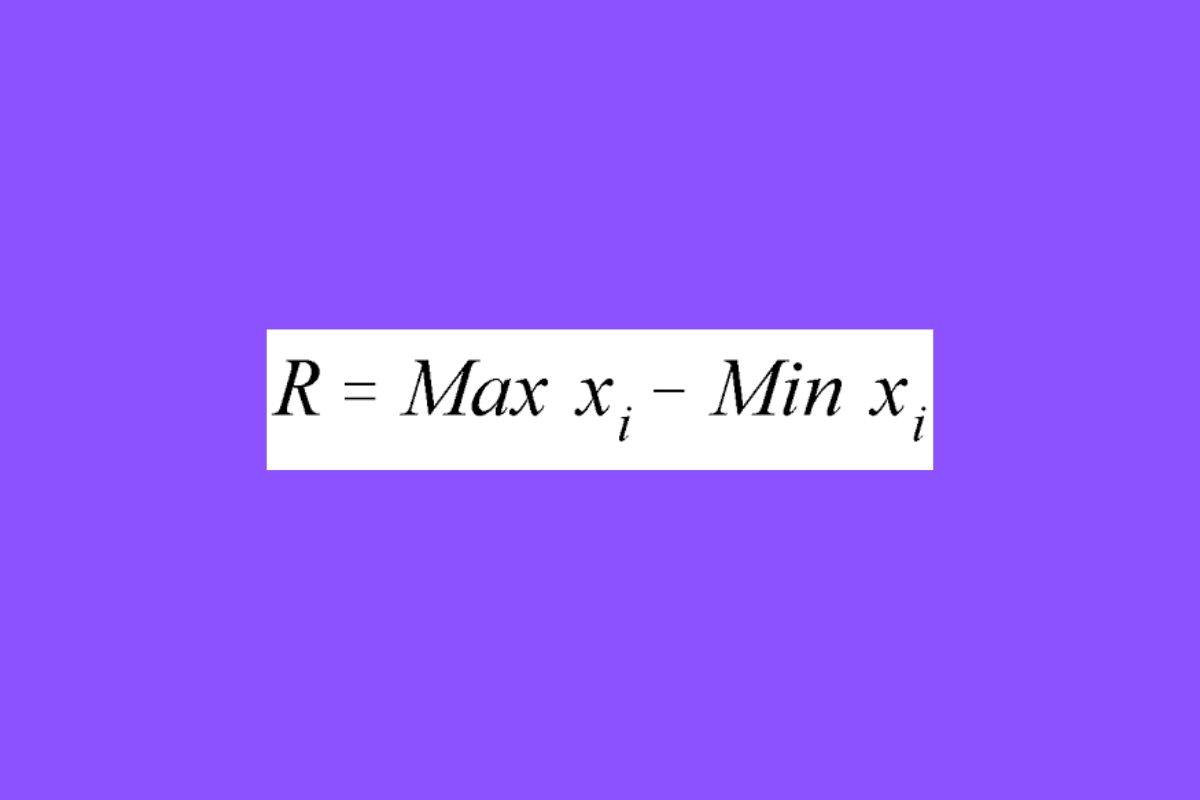For our example of e-commerce customers, we want to know the range of sales:

7,9,10,8,6,6,8,9,7 → 10 – 6 = 4. The rank is 4.

As in the rest of the cases, these are just examples of measures of central tendency, but in case your data volume is much larger than a couple of pizzas and tacos, you can download the free Excel payroll template and automate part of the calculation in a few clicks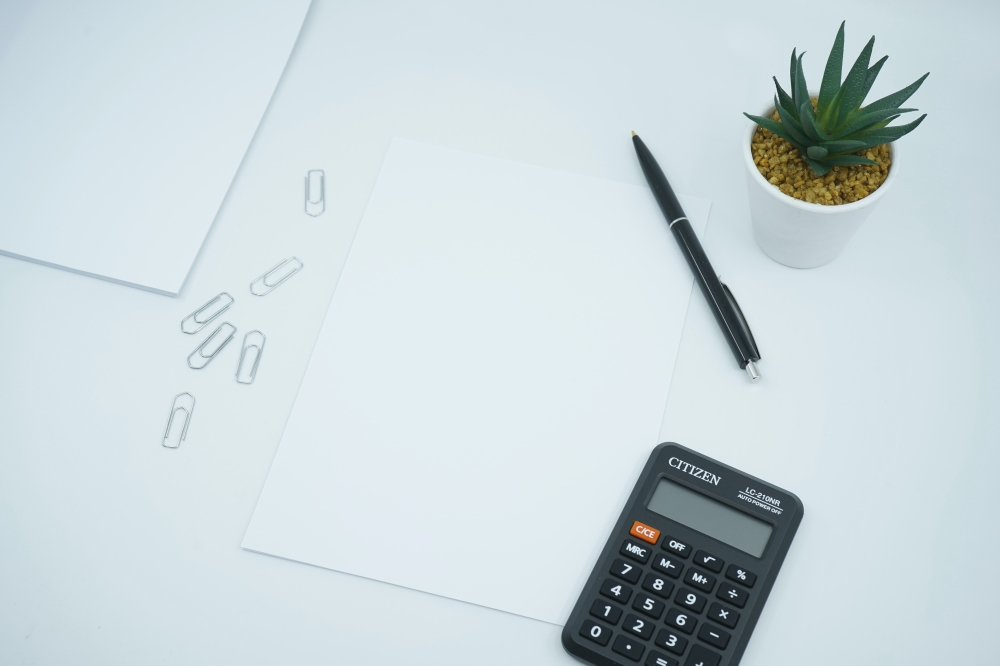Source: Unsplash

You already know all about measures of central tendency. if you want to calculate a very precise average, the mean is the way to go. but it doesn’t mean that the median and mode are useless, they also have their uses, it all depends on the goals you need to accomplish!

Remember that making these calculations is just the beginning, you have all the possibilities to analyze it as you see fit. But the world of data analytics is much bigger than you think. In the meantime, keep practicing with these measures of central tendency.